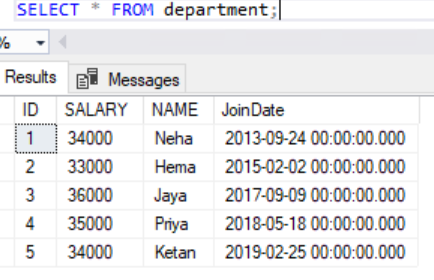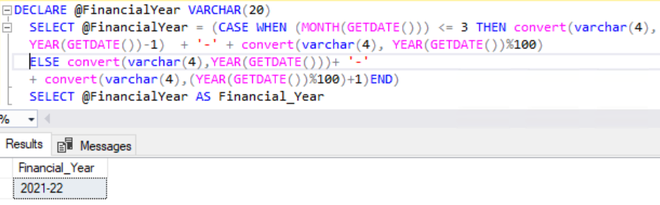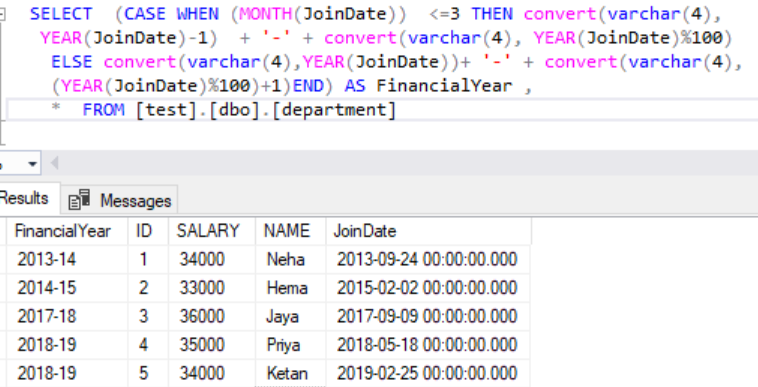# SQL Query to Get a Financial Year Using a Given Date

Here, we are going to  Get a Financial Year Using a Given Date in SQL. In this article, we will be making use of the Microsoft SQL Server as our database.

For example, finding the Financial year for the give dates in the table. Here, we will first create a database named “geeks” then we will create a table “department” in that database. After, that we will execute our query on that table.

### Creating Database:

`CREATE DATABASE geeks;`

### To use this database:

`USE geeks;`

### To create a table in the geeks database:

```CREATE TABLE [dbo].[department](
[ID] [int] NULL,
[SALARY] [int] NULL,
[NAME] [varchar](20) NULL,
[JoinDate] [datetime] NULL
) ON [PRIMARY]
GO```

### Add value into the table:

```INSERT INTO [dbo].[department] VALUES  ( 1, 34000, 'Neha', '2013-09-24')
INSERT INTO [dbo].[department] VALUES  ( 2, 33000, 'Hema', '2015-02-0' )
INSERT INTO [dbo].[department] VALUES  ( 3, 36000, 'Jaya', '2017-09-09' )
INSERT INTO [dbo].[department] VALUES  ( 4, 35000, 'Priya', '2018-05-18' )
INSERT INTO [dbo].[department] VALUES  ( 5, 34000, 'Ketan', '2019-02-25' )
GO```

This is our data inside the table:

`SELECT * FROM department;`To check the current financial year using SQL query:

```DECLARE @FIYear VARCHAR(20)
SELECT @FIYear = (CASE WHEN (MONTH(GETDATE()))
<= 3 THEN convert(varchar(4), YEAR(GETDATE())-1) + '-' + convert(varchar(4), YEAR(GETDATE())%100)
ELSE convert(varchar(4),YEAR(GETDATE()))+ '-' + convert(varchar(4),(YEAR(GETDATE())%100)+1)END)
SELECT @FIYear AS F_YEAR    ```

Output:To Get a Financial Year Using a Given Date in Table:

``` SELECT  (CASE WHEN (MONTH(JoinDate))  <=3 THEN convert(varchar(4),
YEAR(JoinDate)-1)  + '-' + convert(varchar(4), YEAR(JoinDate)%100)
ELSE convert(varchar(4),YEAR(JoinDate))+ '-' + convert(varchar(4),
(YEAR(JoinDate)%100)+1)END) AS FinancialYear ,
*  FROM [test].[dbo].[department]   ```

Output:Unlock the Power of Placement Preparation!
Feeling lost in OS, DBMS, CN, SQL, and DSA chaos? Our Complete Interview Preparation Course is the ultimate guide to conquer placements. Trusted by over 100,000+ geeks, this course is your roadmap to interview triumph.